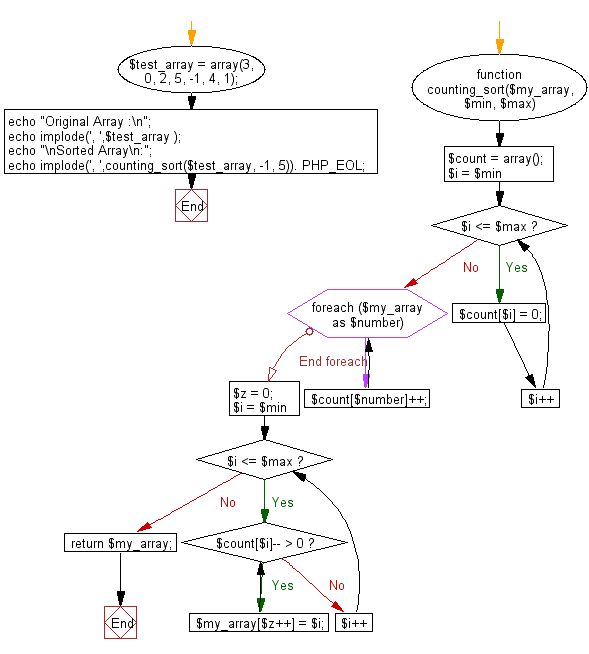# JavaScript Sorting Algorithm: Counting sort

## JavaScript Sorting Algorithm: Exercise-11 with Solution

Write a JavaScript program to sort a list of elements using Counting sort.

According to Wikipedia "In computer science, counting sort is an algorithm for sorting a collection of objects according to keys that are small integers; that is, it is an integer sorting algorithm. It operates by counting the number of objects that have each distinct key value, and using arithmetic on those counts to determine the positions of each key value in the output sequence. Its running time is linear in the number of items and the difference between the maximum and minimum key values, so it is only suitable for direct use in situations where the variation in keys is not significantly greater than the number of items. However, it is often used as a subroutine in another sorting algorithm, radix sort, that can handle larger keys more efficiently".

The algorithm loops over the items, computing a histogram of the number of times each key occurs within the input collection. It then performs a prefix sum computation (a second loop, over the range of possible keys) to determine, for each key, the starting position in the output array of the items having that key. Finally, it loops over the items again, moving each item into its sorted position in the output array.

Sample Solution: -

HTML Code:

``````<!DOCTYPE html>
<html>
<meta charset="utf-8">
<title>JavaScript program of Counting sort</title>
<body></body>
</html>
```
```

JavaScript Code:

``````function countingSort(arr, min, max)
{
var i, z = 0, count = [];

for (i = min; i <= max; i++) {
count[i] = 0;
}

for (i=0; i < arr.length; i++) {
count[arr[i]]++;
}

for (i = min; i <= max; i++) {
while (count[i]-- > 0) {
arr[z++] = i;
}
}
return arr;
}
var arra = [3, 0, 2, 5, 4, 1];
console.log(arra.length);
console.log("Original Array Elements");
console.log(arra);
console.log("Sorted Array Elements");
console.log(countingSort(arra, 0, 5));
```
```

Sample Output:

```6
Original Array Elements
[3,0,2,5,4,1]
Sorted Array Elements
[0,1,2,3,4,5]
```

Flowchart:Live Demo:

See the Pen searching-and-sorting-algorithm-exercise-11 by w3resource (@w3resource) on CodePen.

Improve this sample solution and post your code through Disqus

What is the difficulty level of this exercise?

Test your Programming skills with w3resource's quiz.

﻿